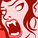# New to Qlik Sense

If you’re new to Qlik Sense, start with this Discussion Board and get up-to-speed quickly.

cancel
Showing results for
Did you mean:Contributor III

## Compare date variable in script

Hello,

I have 4 date variables , iDate, hdate, pDate and paDate. I need to check if all the dates are same.

My code was:

Let vFlag=0;
else
Let vFlag=1;

This doesn't seem to work.

1 Solution

Accepted SolutionsSpecialist II

=if((v1=v2 and v1=v3 and v1=v4 and v2=v3 and v2=v4 and v3=v4 ),1,0)

2 RepliesSpecialist II

=if((v1=v2 and v1=v3 and v1=v4 and v2=v3 and v2=v4 and v3=v4 ),1,0)Contributor III
Author

Thanks!! It works